Editor Ratings:
User Ratings:
[Total: 0 Average: 0]

NUM is a free iPad math game with fun and challenging levels. All the levels in this game include different math problems, which requires you to use your addition, subtraction, multiplication, and division operation to solve them. Each of the mathematical problem differs in the use of these operations, as some of them might get solved with the use of only any one operation while some will require you to apply all the operations.

The game includes a total of 45 levels which you can unlock, as you will go on to clear the levels one by one. The game also lets you replay any level if you fail to solve it, but the app changes the question for the same level.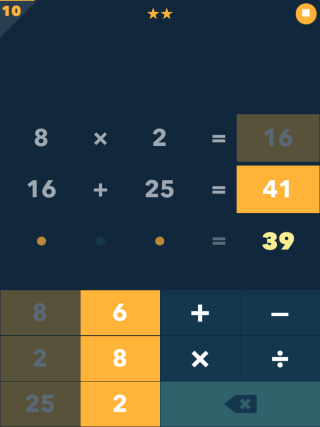## Gameplay of this iPad Math Game NUM:

Since NUM is a iPad math game, so you will have to deal with mathematical problems, but with a twist. Till now, you must be trying to find the answer for mathematical problems, but in this app, you will be finding the mathematical problem after looking at the answer.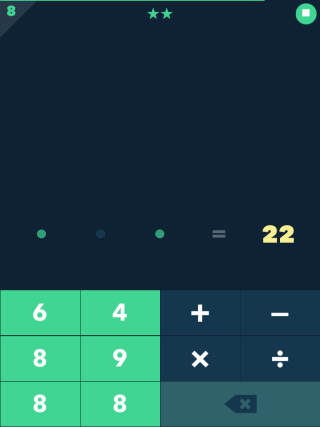For each level, you will see the answer, a few numbers, and the mathematical operations. You have to use at least two numbers and combination of mathematical operation to get the final result given to you already. Sounds easy, isn’t it, but it’s not that easy.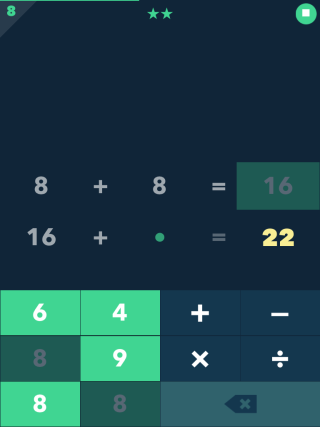The catch in the game is that you can use each number given to you only once. However, there is no restriction on the number of times you use the Mathematical operations. It means that you will have to use the combination of given numbers such that you get the desired solution.

If you give the wrong input, then you can undo the steps easily using the Clear button on the screen. When you complete a level, you proceed to the second level and also earn stars on the basis of time taken by you to solve the problem.In case you fail to answer the question then  you can choose to see the solution, retry the level, or exit to the main menu.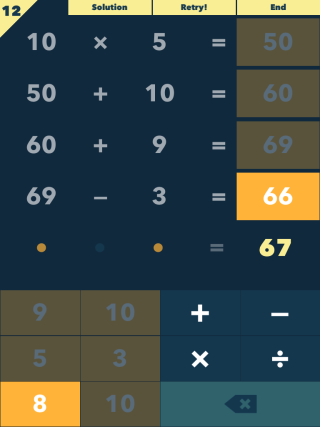The game includes  a total of 45 challenging and fun filled levels to test your Maths skills.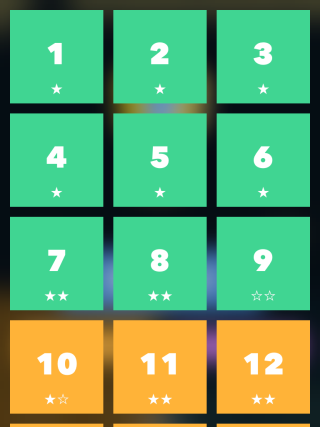You may also like: 5 Free iPhone Math Apps.

## Final Verdict:

NUM is an awesome iPad math game to test your mathematical skills with challenging levels. I enjoyed playing the game completely. The mathematical problems go on to become more and more challenging as you will keep on clearing levels. Do give it a try.